# Hydrostatic pressure is the pressure that is exerted

• Slides: 11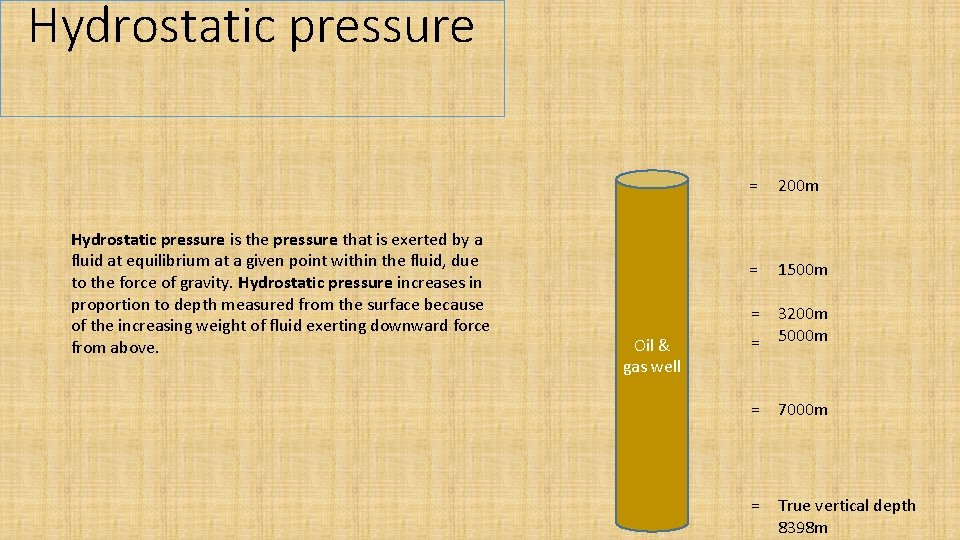Hydrostatic pressure is the pressure that is exerted by a fluid at equilibrium at a given point within the fluid, due to the force of gravity. Hydrostatic pressure increases in proportion to depth measured from the surface because of the increasing weight of fluid exerting downward force from above. Oil & gas well = 200 m = 1500 m = = 3200 m 5000 m = 7000 m = True vertical depth 8398 m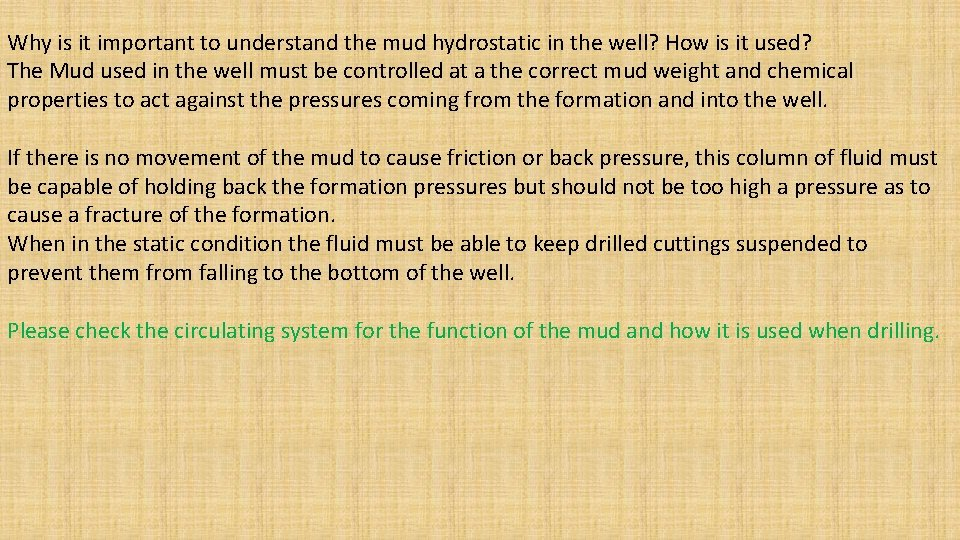Why is it important to understand the mud hydrostatic in the well? How is it used? The Mud used in the well must be controlled at a the correct mud weight and chemical properties to act against the pressures coming from the formation and into the well. If there is no movement of the mud to cause friction or back pressure, this column of fluid must be capable of holding back the formation pressures but should not be too high a pressure as to cause a fracture of the formation. When in the static condition the fluid must be able to keep drilled cuttings suspended to prevent them from falling to the bottom of the well. Please check the circulating system for the function of the mud and how it is used when drilling.Hydrostatic pressure = 200 ft We have to know the hydrostatic pressure in our well at all times. The Hydrostatic pressure is the first safety barrier in the well control systems. To calculate the Hydrostatic pressure in our well we need to know the weight of the fluid in the well and the true vertical depth of the well. We also have to know the constant to use in the calculation. = 1500 ft Oil & gas well = 3200 ft = 5000 ft = 7000 ft = True vertical depth 8398 ftHydrostatic pressure We call the fluid in the well mud. This mud has a weight and is often expressed as MW. For this example the MW will be 9. 6 PPG (pounds per gallon) = 200 ft TVD = 1500 ft TVD MW = 9. 6 ppg Oil & gas well = 3200 ft TVD = 5000 ft TVD = 7000 ft TVD = True vertical depth 8398 ftHydrostatic pressure The constant used for this example is 0. 052 this constant will be used when ever we work out the MW in ppg. = 200 ft TVD = 1500 ft TVD MW = 9. 6 ppg Constant = 0. 052 Oil & gas well = 3200 ft TVD = 5000 ft TVD = 7000 ft TVD = True vertical depth 8398 ftHydrostatic pressure This element of the formula is the True Vertical Depth or TVD and we can Calculate the hydrostatic at any point in the well using the TVD The important thing to understand is that the depth could be 11000 ft as there could be deviations from vertical. If we are talking about Pressure we need to know TVD , remember this. MW = 9. 6 ppg Constant = 0. 052 TVD = 8398. Oil & gas well = 200 ft TVD = 1500 ft TVD = = 3200 ft TVD 5000 ft TVD = 7000 ft TVD = True vertical depth 8398 ftHydrostatic pressure Here is an example of how to use the formula. = 200 ft TVD = 1500 ft TVD = = 3200 ft TVD 5000 ft TVD = 7000 ft TVD = True vertical depth 8398 ft MW 9. 6 x constant 0. 052 x TVD 8398 = 4192 psi (pounds per square inch) 9. 6 x. 052 x 8398= 4192 psi. Oil & gas well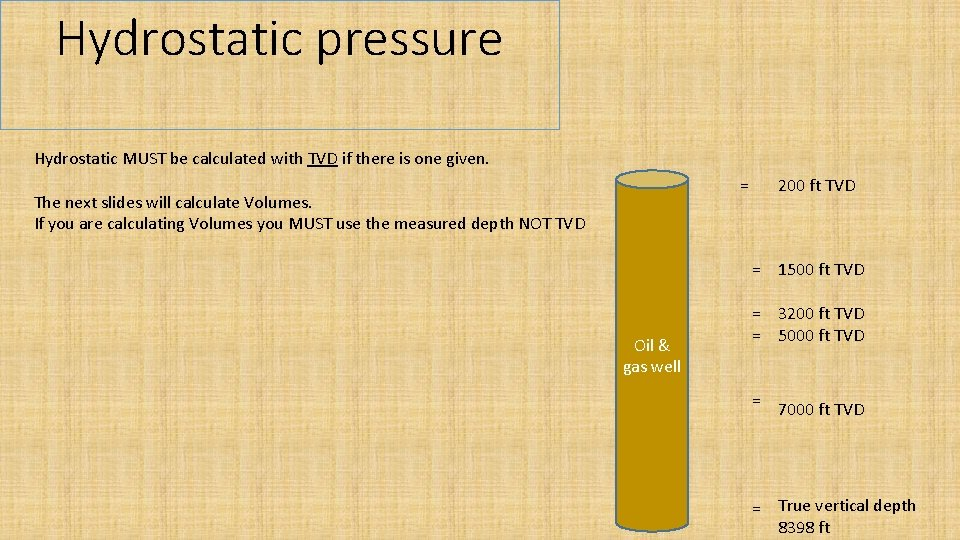Hydrostatic pressure Hydrostatic MUST be calculated with TVD if there is one given. = The next slides will calculate Volumes. If you are calculating Volumes you MUST use the measured depth NOT TVD 200 ft TVD = 1500 ft TVD Oil & gas well = 3200 ft TVD = 5000 ft TVD = 7000 ft TVD = True vertical depth 8398 ft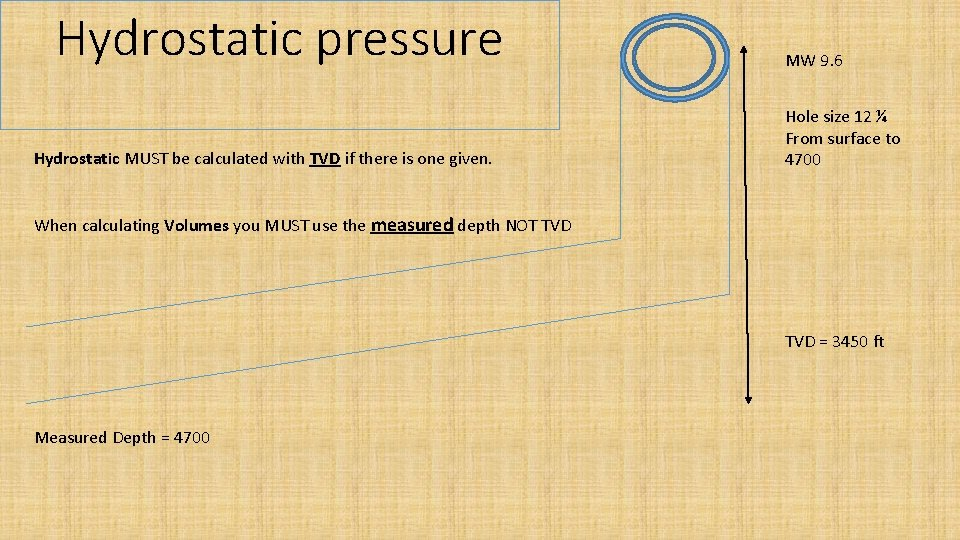Hydrostatic pressure Hydrostatic MUST be calculated with TVD if there is one given. MW 9. 6 Hole size 12 ¼ From surface to 4700 When calculating Volumes you MUST use the measured depth NOT TVD = 3450 ft Measured Depth = 4700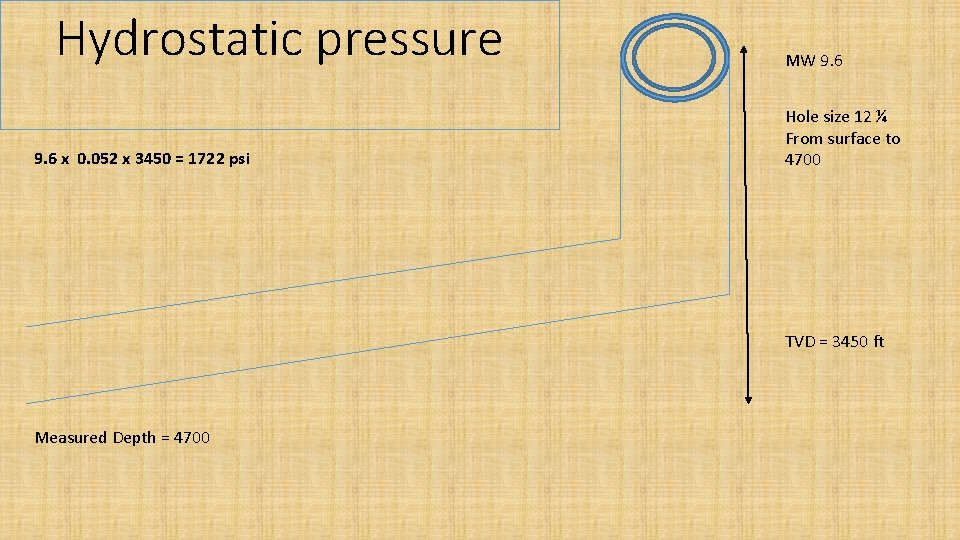Hydrostatic pressure 9. 6 x 0. 052 x 3450 = 1722 psi MW 9. 6 Hole size 12 ¼ From surface to 4700 TVD = 3450 ft Measured Depth = 4700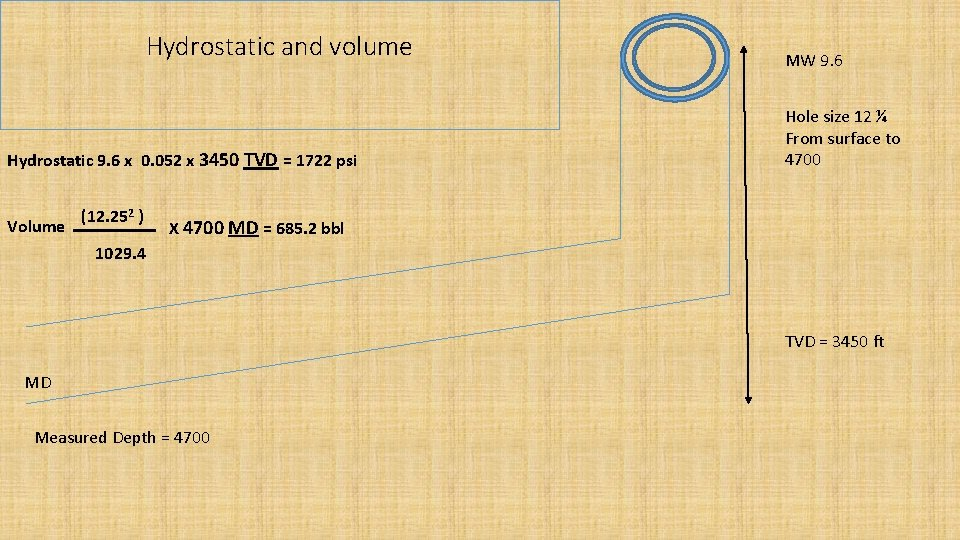Hydrostatic and volume Hydrostatic 9. 6 x 0. 052 x 3450 TVD = 1722 psi Volume (12. 252 ) MW 9. 6 Hole size 12 ¼ From surface to 4700 X 4700 MD = 685. 2 bbl 1029. 4 TVD = 3450 ft MD Measured Depth = 4700